Create a new printableMath Worksheets
Time

Sample - Click above to make a new math worksheet (PDF).
 Name _____________________________Date ___________________
Time
Write the time two ways.
1.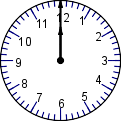___ o'clock___ : ___
 2 * This is a pre-made sheet.Use the link at the top of the page for a printable page.
3.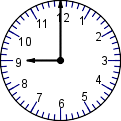___ o'clock___ : ___

Write the time two ways.
4.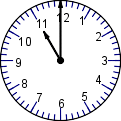___ o'clock___ : ___
5.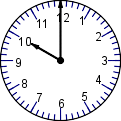___ o'clock___ : ___
6.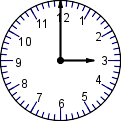___ o'clock___ : ___
7.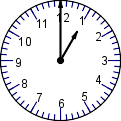___ o'clock___ : ___

Write the time.
8.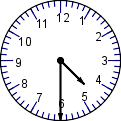half past ___
9.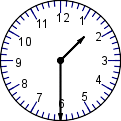half past ___
10.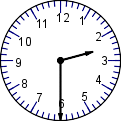half past ___

 Key #2
 aKey #2
Write the time two ways.
11.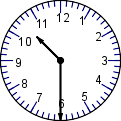half past ______ : ___
12.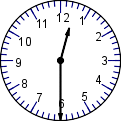half past ______ : ___
13.half past ______ : ___
14.half past ______ : ___

Write the time two ways.
15.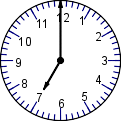___ o'clock___ : ___
16.___ o'clock___ : ___
17.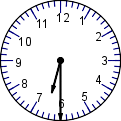half past ______ : ___

Write the time.
18.___ : ___
19.___ : ___
20.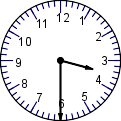___ : ___
21.___ : ___

Draw the two hands on the clock to show the time.
22.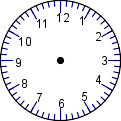2:00
23.8:30
24.7:00
25.11:30